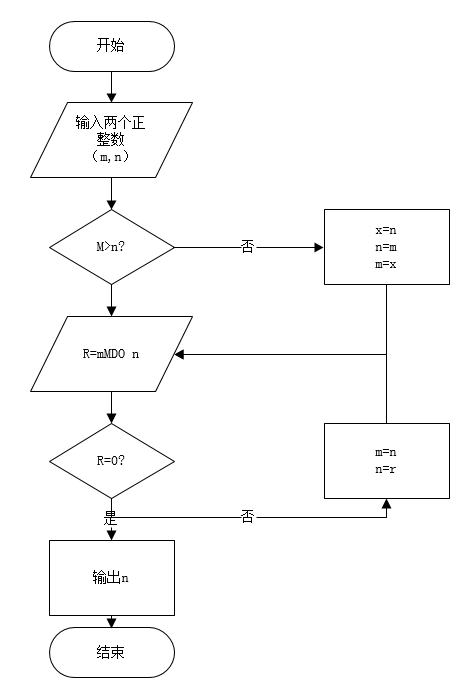•闪存
•博客
• 发言小组
• 投递新闻
• 提问博问
• 添加收藏
•文库

0悬赏园豆：10 [已解决问题] 解决于 2019-10-16 20:04# include<stdio.h>

int main(void)
{int a,b,t,mult;
char ch;
scanf("%d%c%d",&a,&ch,&b);//自己想的是把空格的值赋值给ch
mult=ab;
if(a<b)
{a=t;
a=b;
b=t;
}
while(b!=0) //求最大公约数
{t=a%b;
a=b;
b=t;
}
printf("%d\n",a);
printf("%d\n",mult/a);//最小公倍数==（a
b）/最大公约数
return 0;
}

1# include <stdlib.h>

int main()
{
int m,n,a,b,t,temp,h;
printf("输入m和n\n");
scanf_s("%d%d", &m, &n);
a=m;b=n;
if(a<b)
{
t=a;a=b;b=t;
}
while(b!=0) //求最大公约数
{
temp=a%b;a=b;b=temp;
}
h=m*n/a;//求最小公倍数
printf("%d和%d的最大公约数是:%d\n",m,n,a);
printf("%d和%d的最小公倍数是:%d\n",m,n,h);
system("pause");
}

emmm，我写的代码用的思想几乎跟你这个相似，自己输入一些数，得到的结果也和预想的一样，但oj

Fate-Joker | 园豆：158 (初学一级) | 2019-10-15 19:28

Fate-Joker | 园豆：158 (初学一级) | 2019-10-16 20:03

您需要登录以后才能回答，未注册用户请先注册